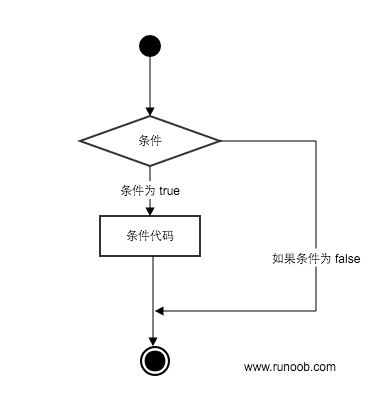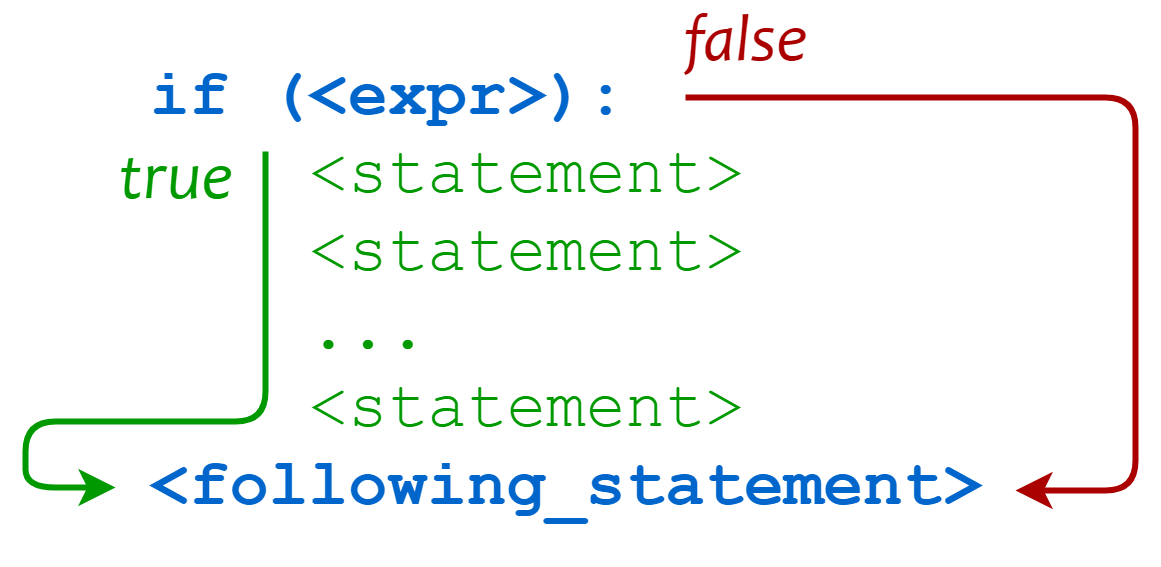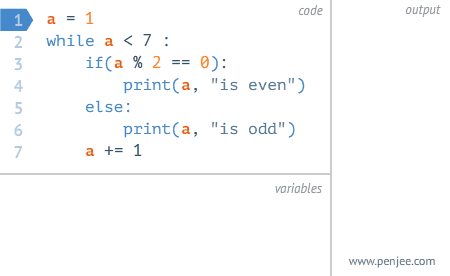# Python3基础之Python3条件控制

Python3.X作者：顾小北日期：2021-04-28点击：179

Python 条件语句是通过一条或多条语句的执行结果（True 或者 False）来决定执行的代码块。## if 语句

Python中if语句的一般形式如下所示：

if condition_1: statement_block_1 elif condition_2: statement_block_2 else: statement_block_3
• 如果 "condition_1" 为 True 将执行 "statement_block_1" 块语句
• 如果 "condition_1" 为False，将判断 "condition_2"
• 如果"condition_2" 为 True 将执行 "statement_block_2" 块语句
• 如果 "condition_2" 为False，将执行"statement_block_3"块语句

Python 中用 elif 代替了 else if，所以if语句的关键字为：if – elif – else

• 1、每个条件后面要使用冒号 :，表示接下来是满足条件后要执行的语句块。
• 2、使用缩进来划分语句块，相同缩进数的语句在一起组成一个语句块。
• 3、在Python中没有switch – case语句。

Gif 演示：## 实例

#!/usr/bin/python3 var1 = 100 if var1: print ("1 - if 表达式条件为 true") print (var1) var2 = 0 if var2: print ("2 - if 表达式条件为 true") print (var2) print ("Good bye!")

```1 - if 表达式条件为 true
100
Good bye!
```

## 实例

#!/usr/bin/python3 age = int(input("请输入你家狗狗的年龄: ")) print("") if age <= 0: print("你是在逗我吧!") elif age == 1: print("相当于 14 岁的人。") elif age == 2: print("相当于 22 岁的人。") elif age > 2: human = 22 + (age -2)*5 print("对应人类年龄: ", human) ### 退出提示 input("点击 enter 键退出")

```\$ python3 dog.py

```

`<` 小于
`<=` 小于或等于
`>` 大于
`>=` 大于或等于
`==` 等于，比较两个值是否相等
`!=` 不等于

## 实例

#!/usr/bin/python3 # 程序演示了 == 操作符 # 使用数字 print(5 == 6) # 使用变量 x = 5 y = 8 print(x == y)

```False
False
```

high_low.py文件演示了数字的比较运算：

## 实例

#!/usr/bin/python3 # 该实例演示了数字猜谜游戏 number = 7 guess = -1 print("数字猜谜游戏!") while guess != number: guess = int(input("请输入你猜的数字：")) if guess == number: print("恭喜，你猜对了！") elif guess < number: print("猜的数字小了...") elif guess > number: print("猜的数字大了...")

```\$ python3 high_low.py

```

## if 嵌套

```if 表达式1:
语句
if 表达式2:
语句
elif 表达式3:
语句
else:
语句
elif 表达式4:
语句
else:
语句
```

## 实例

# !/usr/bin/python3 num=int(input("输入一个数字：")) if num%2==0: if num%3==0: print ("你输入的数字可以整除 2 和 3") else: print ("你输入的数字可以整除 2，但不能整除 3") else: if num%3==0: print ("你输入的数字可以整除 3，但不能整除 2") else: print ("你输入的数字不能整除 2 和 3")

```\$ python3 test.py

```